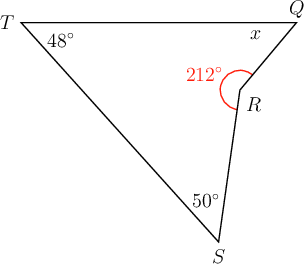Home Practice
For learners and parents For teachers and schools
Textbooks
Full catalogue
Pricing SupportLog in

We think you are located in United States. Is this correct?

# End of chapter exercises

## End of chapter exercises

temp text

### Calculate complementary and supplementary angles

1. Given line segment $$MN = 10 \text{ cm}$$.

1. Construct $$PQ$$, the perpendicular bisector of $$MN$$.
2. Label point $$R$$, the point of intersection of $$MN$$ and $$PQ$$.
3. Measure and confirm that $$MR = RN$$.
2. The diagrams below show the construction of a perpendicular bisector. The order of the diagrams is not correct. Study the diagrams and write down the correct order for constructing a perpendicular bisector of $$AB$$.

• Diagram 1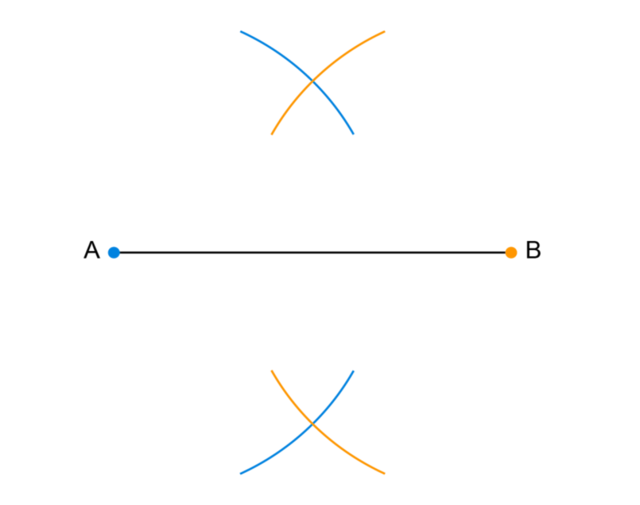• Diagram 2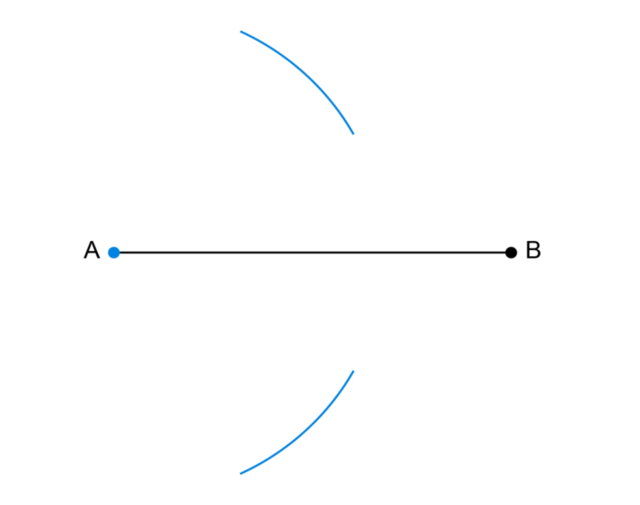• Diagram 3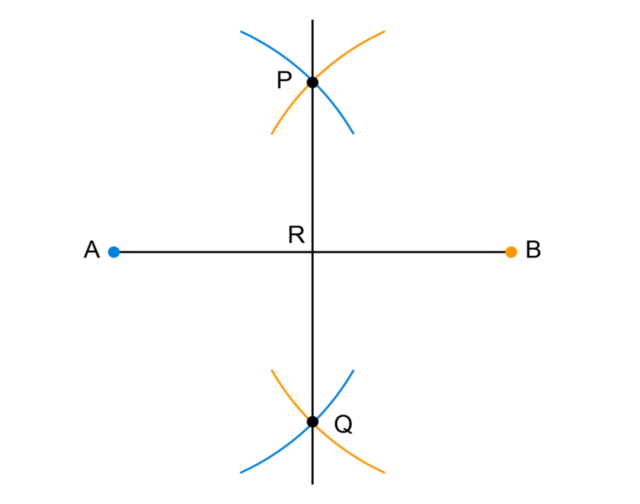• Diagram 4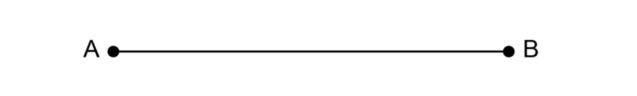3. Draw $$\triangle ABC$$. Choose any length for the sides of $$\triangle ABC$$.

1. Construct the perpendicular bisector of $$AB$$.
2. Construct the perpendicular bisector of $$BC$$.
3. What do you notice about these two lines?
4. Construct the perpendicular bisector of $$CA$$.
4. Use a ruler and a compass to construct $$\triangle DEF$$ with $$DE = 10 \text{ cm}$$, $$EF = 8 \text{ cm}$$ and $$FD = 6 \text{ cm}$$.

5. Show that the sum of interior angles of a rectangle is $$360^{\circ}$$.

6. Use a ruler and a compass to construct $$\triangle STU$$ with $$ST = 5 \text{ cm}$$, $$TU = 4 \text{ cm}$$ and $$US = 3 \text{ cm}$$.

1. What is the complement of $$28^{\circ}$$?
2. What is the complement of $$70^{\circ}$$?
3. What is the supplement of $$62^{\circ}$$?
4. What is the supplement of $$103^{\circ}$$?
8. Use a ruler and a compass to construct equilateral $$\triangle ABC$$ with $$AB = 7 \text{ cm}$$.

9. Use a compass and a ruler to construct square $$JKLM$$ with $$JK = \text{6,5} \text{ cm}$$.

10. Show that the sum of interior angles of a square is $$360^{\circ}$$.

11. In quadrilateral $$QRST$$ below, $$\hat{Q} = 84^{\circ}, \hat{S} = 105^{\circ}$$ and $$\hat{T} = 65^{\circ}$$. Determine the value of $$x$$, giving a reason for your answer.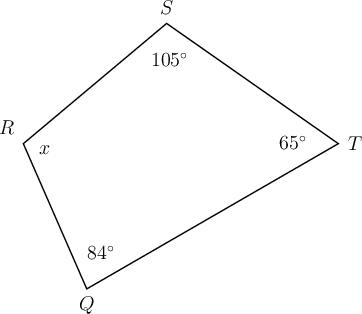12. In quadrilateral $$QRST$$is given. $$\hat{R} = 212^{\circ}, \hat{S} = 50^{\circ}$$ and $$\hat{T} = 48^{\circ}$$. Find the value of $$x$$, giving a reason for your answer.